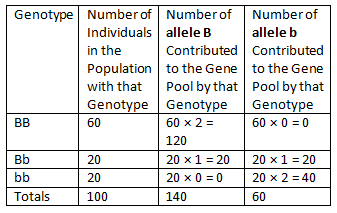# ACT Science Practice Test 3

Directions: The passage below is followed by several questions. After reading the passage, choose the best answer to each of the ACT Science practice test questions. You may refer to the passage as often as necessary. Calculators may NOT be used.

Passage III

Allele Frequencies

Allele frequency is how often an allele occurs in a gene pool relative to the other alleles for that gene. Look at the example in Table 1 below. The population in the table has 100 members. In a sexually reproducing species, each member of the population has two copies of each gene. Therefore, the total number of copies of each gene in the gene pool is 200. The gene in the example exists in the gene pool in two forms, alleles A and a. Knowing the genotypes of each population member, we can count the number of alleles of each type in the gene pool. The table shows how this is done.Let the letter p stand for the frequency of allele A. Let the letter q stand for the frequency of allele a. We can calculate p and q as follows:

p = number of A alleles/total number of alleles = 140/200 = 0.7

q = number of a alleles/total number of alleles = 60/200 = 0.3

Notice that p + q = 1.

Evolution occurs in a population when allele frequencies change over time. What causes allele frequencies to change? That question was answered by Godfrey Hardy and Wilhelm Weinberg in 1908.

Hardy and Weinberg hypothesized that when certain conditions are met, allele frequencies stay the same. Genotype frequencies also remain constant. In addition, genotype frequencies can be expressed in terms of allele frequencies, as Table 2 below shows.Hardy and Weinberg used mathematics to describe an equilibrium population (p = frequency of A, q = frequency of a): p2 + 2pq + q2 = 1.

Tables and passage excerpted from CK-12 Foundation, Biology. Copyright © 2012 by CK-12 Foundation.

Congratulations - you have completed . You scored %%SCORE%% out of %%TOTAL%%. Your performance has been rated as %%RATING%%
 Question 1
For the population described in Table 1, how many type A alleles are contributed to the gene pool by genotype Aa?

 A 20 B 40 C 60 D 100
Question 1 Explanation:
The correct answer is (B). If you read Table 1 correctly, you should see that the cell at the point of intersection of the third row and the third column in Table 1 contains the needed information. 40 type A alleles are contributed to the gene pool by genotype Aa.
 Question 2
Based on Table 1, what is the frequency of Allele a?

 A 0.25 B 0.3 C 0.4 D 0.7
Question 2 Explanation:
The correct answer is (B). The frequency of allele a is calculated and reported right below Table 1. The frequency of allele a is q.
q = number of a alleles/total number of alleles
= 60/200
= 0.3
 Question 3
Based on the information presented, evolution will occur in a population under which of the following conditions?

 A When the number of alleles in the population changes over time. B When individuals do not choose mates based on genotypes. C When all members of the population have an equal chance of reproducing and passing their genes to the next generation. D When the proportion of a given allele relative to the other alleles in the population changes over time.
Question 3 Explanation:
The correct answer is (D). We are told that “Evolution occurs in a population when allele frequencies change over time.” Answer choice (A) is incorrect because if the number of alleles in a population changes but the proportion of that allele stays the same, then the frequency of that allele will stay the same because the “frequency is how often an allele occurs in a gene pool relative to the other alleles for that gene”.
 Question 4
Based on Table 2, if q = 0.4, what is the frequency of the aa genotype?

 A 0.16 B 0.36 C 0.4 D 0.48
Question 4 Explanation:
The correct answer is (A). Based on Table 2, the frequency of the aa genotype is q². Therefore, if q = 0.4, the frequency of the aa genotype = 0.4² = 0.16.
 Question 5
If a population of 100 individuals has the following numbers of genotypes for a gene with two alleles, B and b: BB = 60, Bb = 20, and bb = 20, what is the frequency of allele B based on the table below?A 0.3 B 0.6 C 0.7 D 1.4
Question 5 Explanation:
The correct answer is (C). The frequency of allele B is 0.7.

Let the letter p stand for the frequency of allele B. Let the letter q stand for the frequency of allele b. We can calculate p and q as follows:

p = number of B alleles/total number of alleles
= 140/200
= 0.7

q = number of b alleles/total number of alleles
= 60/200
= 0.3
Once you are finished, click the button below. Any items you have not completed will be marked incorrect.
There are 5 questions to complete.
 ← List →